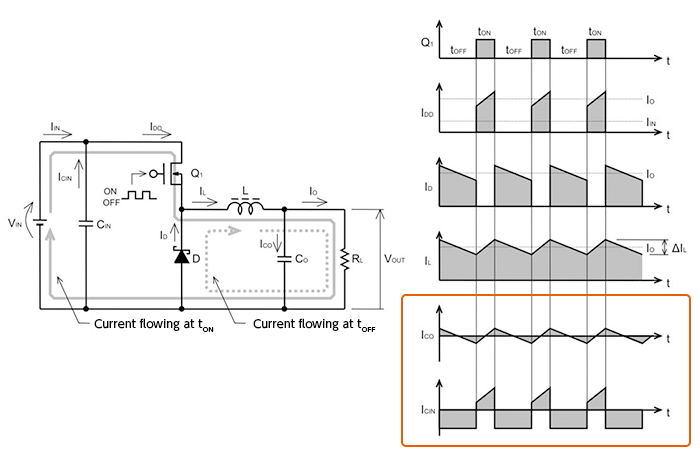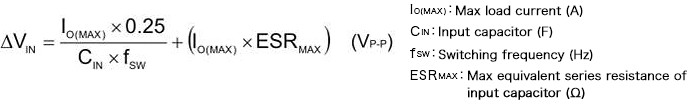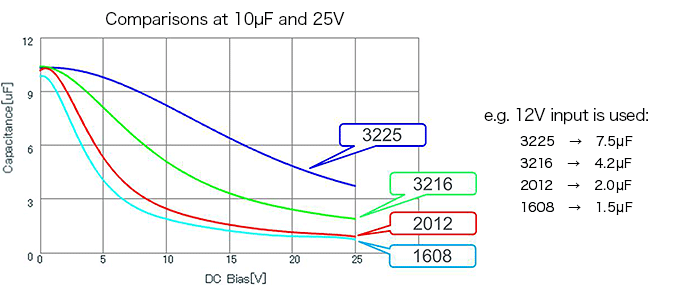# Selection of Input Capacitors

2017.06.08

・Important issues in capacitor selection include the rated voltage, rated ripple current, characteristic for heat generation by ripples, and when using a ceramic capacitor, the temperature characteristic and DC bias characteristic in particular.

・Input capacitors must be able to tolerate higher voltages and currents than output capacitors.

In the preceding section, we explained the role of output capacitors and important points in their selection. Next, we turn to an explanation of input capacitors. The following diagrams were also used in the section of output capacitors, but to aid understanding we use them again in an initial review of capacitor operation.

## The Roles of Input and Output Capacitors

As in the previous section, we review the flow of currents in a DC-DC converter, and consider the nature of the currents flowing in the input and output capacitors.In the waveforms enclosed in the rectangle, the upper waveform ICO and the lower waveform ICIN are the current waveforms of the output and input capacitors respectively. The input capacitor is charged by VIN, and when the transistor Q1 turns on, discharges a current which becomes the switch current IDD. Comparatively high currents flow suddenly and repeatedly. In contrast, the output capacitor is repeatedly charged and discharged according to the output ripple voltage, which is centered on the output voltage; this difference should be kept in mind.

## Selection of the input capacitor

The following three parameters are important when selecting the input capacitor.

1） Rated voltage

2） Rated ripple current and ripple heat generation characteristics

3） When using a ceramic capacitor: temperature characteristic and DC bias characteristic

Moreover, the following should be born in mind as premises for selection.

・The rated voltage must be higher than the maximum input voltage.

・The rated ripple current must be greater than the maximum input ripple current occurring in the IC input.

・In a step-down converter, the maximum value of the instantaneous input current is the same as the output current.

The effective value ICIN of the ripple current flowing in the input capacitor is represented by the following equation.Based on this result, and using the absolute maximum rating of ripple current and a graph of the ripple heat generation characteristic, a capacitor with the required performance is selected.

The input ripple voltage ΔVIN can be calculated as follows.From this equation, we see that the input ripple voltage is smaller for larger values of the capacitance of the input capacitor.

A ceramic capacitor can be selected as an input capacitor. When using a ceramic capacitor, attention must generally be paid to temperature changes and to changes in capacitance due to the DC bias.

Where temperature characteristics are concerned, if the capacitor is a Class 2 (high dielectric constant) type, with an EAI code of X5R (-55 to +85°C, electrostatic capacitance changing rate ±15%) or X7R (-55 to +125°C, capacitance changing rate ±15%), then a sufficiently stable temperature characteristic can be obtained.

Where DC bias is concerned, of course a capacitor that is not greatly affected should be selected, but even for the same capacitance and voltage, fluctuation characteristics differ depending on the package size. The following graph illustrates some examples; capacitors with larger sizes exhibit less fluctuation. In any case, thorough information should be obtained from the capacitor manufacturer.In essence, the input capacitor is selected on the basis of these parameters, but in trial manufacture and evaluation, checks must be performed to ensure that the input voltage with ripples added do not exceed the withstand voltage, and that heat generation caused by the ripple current can be tolerated.